# True Stress – True Strain Curve: Part Four

This article describes strain-hardening exponent and strength coefficient, materials constants which are used in calculations for stress-strain behaviour in work hardening, and their application in some of the most commonly used formulas, such as Ludwig equation.

The flow curve of many metals in the region of uniform plastic deformation can be expressed by the simple power curve relation

(10)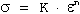where n is the strain-hardening exponent and K is the strength coefficient. A log-log plot of true stress and true strain up to maximum load will result in a straight-line if Eq. (10) is satisfied by the data (Fig. 1).

The linear slope of this line is n and K is the true stress at e = 1.0 (corresponds to q = 0.63). The strain-hardening exponent may have values from n = 0 (perfectly plastic solid) to n = 1 (elastic solid), see Fig. 2. For most metals n has values between 0.10 and 0.50, see Table 1.

It is important to note that the rate of strain hardening ds /de, is not identical with the strain-hardening exponent. From the definition of n

(11)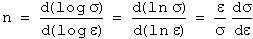or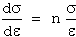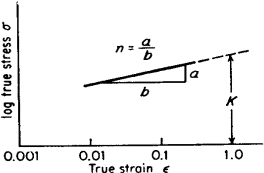Figure 2. Log/log plot of true stress-strain curveFigure 3. Various forms of power curve s=K* e n

Table 1. Values for n and K for metals at room temperatureThere is nothing basic about Eq. (10) and deviations from this relationship frequently are observed, often at low strains (10-3) or high strains (e»1,0).

One common type of deviation is for a log-log plot of Eq. (10) to result in two straight lines with different slopes. Sometimes data which do not plot according to Eq. (10) will yield a straight line according to the relationship

(12)Datsko has shown how e0, can be considered to be the amount of strain hardening that the material received prior to the tension test.

Another common variation on Eq. (10) is the Ludwig equation

(13)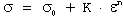where s0 is the yield stress and K and n are the same constants as in Eq. (10). This equation may be more satisfying than Eq. (10) since the latter implies that at zero true strain the stress is zero.

Morrison has shown that s0 can be obtained from the intercept of the strain-hardening point of the stress-strain curve and the elastic modulus line by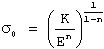The true-stress-true-strain curve of metals such as austenitic stainless steel, which deviate markedly from Eq. (10) at low strains, can be expressed by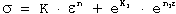where eK is approximately equal to the proportional limit and n1 is the slope of the deviation of stress from Eq. (10) plotted against e. Still other expressions for the flow curve have been discussed in the literature.

The true strain term in Eqs.(10) to (13) properly should be the plastic strain

ep= etotal– eE= etotal– s/E